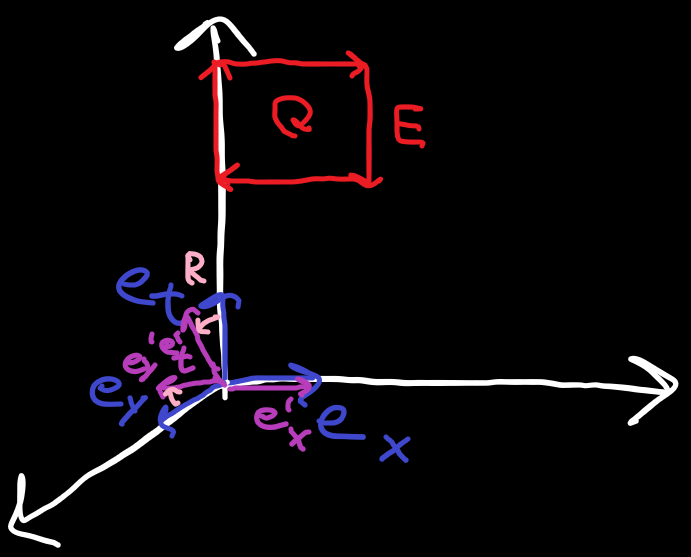### Special Relativity with Geometric Algebra - Electromagnetism

Electromagnetism is most often described using Maxwell's equations in vector form. In Geometric Algebra these can be expressed as a single equation and a field that unifies the electric and magnetic fields. This also makes it very easy to combine our results from Special Relativity with Electromagnetism.
There are already a lot of resources showing how to do Electromagnetism with GA. Here are a couple of short ones that get the essence if you are interested:
For now we actually won't use any of Maxwell's equations. The relevant part to us is that the electric and magnetic fields will be bivector fields$E$and$B$using the bivectors that square to$+1$(${e}_{tx},{e}_{ty},{e}_{tz}$corresponding to each direction in space) and that they can be combined into a single bivector field$F$called the Faraday field in the following way:
$\begin{array}{}\text{(1)}& F=E+IB\end{array}$
Because the magnetic bivector field is multiplied by the pseudoscalar$I$, in general$F$contains all bivectors in the algebra.
In the next part we will investigate an electric field as seen by an observer moving orthogonal to it.

#### Electric field seen by observer moving orthogonal to itFigure 1 - Blue: Rest frame. Purple: Moving observer's frame. Red: Electric field in x direction of rest frame. Pink: Rotor from rest frame to moving observer's frame.
Let's start with an electric field$E$with only a value${E}_{x}$in the$x$direction
$\begin{array}{}\text{(2)}& F=E={E}_{x}{e}_{tx}\end{array}$
The basis vectors for an observer moving relative to the field with rapidity${\phi }_{y}$in the$y$direction can be obtained with a passive transformation by applying a rotor to the basis vectors at rest.
$\begin{array}{}\text{(3)}& {R}_{y}\left({\phi }_{y}\right)={e}^{-\frac{{\phi }_{y}}{2}{e}_{ty}}\end{array}$
The rotor${R}_{y}$transforms from the rest frame to the moving observer's frame. We can now measure the field with the reciprocal basis vectors of the moving frame to figure out how a moving observer sees the field.
Before continuing with the algebra, let's take another look at the diagram. What would we expect to happen if we applied the rotor to the field? The bivector field lies in the TX plane. The rotor (hyperbolically) rotates between the T and the Y axis. So we would expect the resulting bivector field to have not only have a TX component but also an XY component.
We can also see this visually: the original XY plane was orthogonal to$E$'s plane, but the XY' plane is not orthogonal anymore.
##### Reciprocal basis bivectors
Okay now for the algebra: because we are measuring bivectors and not vectors, we want to build reciprocal bivectors. For example to measure the XY component, we have a basis bivector${e}_{xy}$and its corresponding reciprocal basis bivector is${e}^{xy}=-{e}_{xy}$because$-{e}_{xy}\cdot {e}_{xy}=1$.
We already established that the moving observer should see a field in the TX and the XY plane, so let's build the reciprocal basis bivectors for those.
$\begin{array}{}\text{(4)}& \begin{array}{rl}{{e}^{tx}}^{\prime }& ={R}_{y}{e}^{tx}{\stackrel{~}{R}}_{y}={R}_{y}{e}_{tx}{\stackrel{~}{R}}_{y}=\mathrm{cosh}\left({\phi }_{y}\right){e}_{tx}+\mathrm{sinh}\left(\phi \right){e}_{xy}\\ {{e}^{xy}}^{\prime }& ={R}_{y}{e}^{xy}{\stackrel{~}{R}}_{y}=-{R}_{y}{e}_{xy}{\stackrel{~}{R}}_{y}=-\left(\mathrm{cosh}\left({\phi }_{y}\right){e}_{xy}+\mathrm{sinh}\left(\phi \right){e}_{tx}\right)\end{array}\end{array}$
##### Field measured by orthogonally moving observer
Taking the inner product of the field with the reciprocal basis bivectors yields
$\begin{array}{}\text{(5)}& \begin{array}{rl}{F}_{tx}^{\prime }& ={{e}^{tx}}^{\prime }\cdot F={E}_{x}\mathrm{cosh}\left({\phi }_{y}\right)\\ {F}_{xy}^{\prime }& ={{e}^{xy}}^{\prime }\cdot F=-{E}_{x}\mathrm{sinh}\left({\phi }_{y}\right)\end{array}\end{array}$
We can see the the TX component of the field gets stronger the faster the observer moves in the Y direction (both positive and negative). We also have an XY component. If we look back to how$F$was defined we can see that this part comes from$IB$, specifically the Z component of the magnetic field because${e}_{xy}=I{e}_{tz}$. So a moving observer will also see a magnetic field perpendicular to both their movement direction and the electric field's direction.
$\begin{array}{}\text{(6)}& \begin{array}{rl}{E}_{x}^{\prime }& ={E}_{x}\mathrm{cosh}\left({\phi }_{y}\right)\\ {B}_{z}^{\prime }& =-{E}_{x}\mathrm{sinh}\left({\phi }_{y}\right)\end{array}\end{array}$

#### Conclusion

We saw that the electromagnetic field is described by the faraday bivector field$F=E+IB$. Then we looked at how an observer moving orthogonal to an electric field sees the field. We observed that the original electric field gets stronger, but we also saw that the observer measures a magnetic field in the direction orthogonal to both the movement direction and the original electric field.